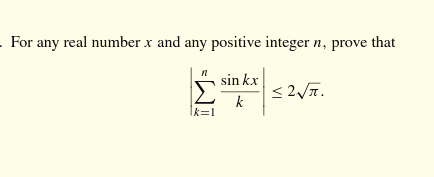# Doubt from trignometry1 Like

It resembles Fejer's inequality but cant find a Wiki link to it. Give me some time

Sir whenever free kindly share your approach
I am trying but not getting idea

@Sneha_2021 what is the source?

Are you talking of this??

• Sir its concept out of my mind can you explain it little bit??

No idea some one asked me @Samrat_2020

euclid.ijm.1258131058.pdf (248.8 KB)

1 Like

These all solution dont giving idea about upper bound why only it should be less then {2\sqrt{\pi}}

\displaystyle \sum_{k=1}^n \left(\frac{sin{kx}}{k}\right) is a Fourier series of a sawtooth wave

I tried to find a Fourier series of a sawtooth wave but that doesn't go good
All I get that the amplitude of sawtooth wave here is 1

1 Like

I found the function
\frac{π-x}{2}+π[\frac{x}{2π}]
For 0<x<π

[•] is GIF

This one is provable if you take that sine function to be an like this